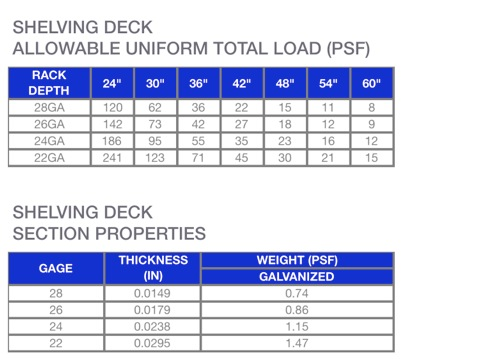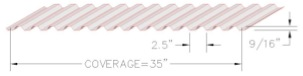Example Calculation:
A shelving level is 24” deep x 96” wide. What would be the lightest gage of shelving deck allowable to achieve a uniform storage level capacity of 2,000 pounds (LBS)?

Step 1 Determine the area (SF) of the storage level
24” x 96” = 2,304 SQ IN = 16 SF

Step 2 Divide the required capacity (2,000 LBS) by the area of the storage level (16 SF) to obtain the total load
2000 LBS/16 SF = 125 LBS/SF = 125 PSF

Step 3 Find the lowest number above 125 PSF in the 24” column and match the gage

Solution: 142 PSF = 26GA SHELVING DECK